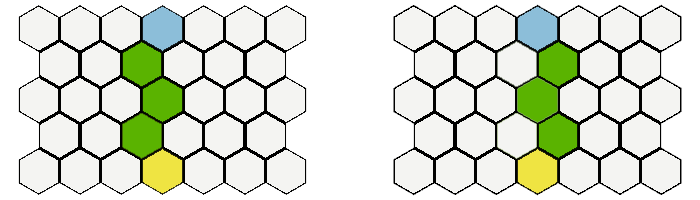HexMapLibrary
Example 2: Line of sight

In this Example we want to create a simple vision system. If we click on a tile we want to highlight all those tiles which are visible. There will be 2 rules:
1) there is a limited vision range
2) certain tiles block vision to anything behind them

To decide which tiles are visible we are going to use the following approach: We draw a ring around the center tile and then draw lines to each tile of the ring, stopping each line after it hit a vision blocker. Every tile which at least one line reaches is considered visible. As we use the ring just as a helper to create the lines we want to also get those ring tiles which are out of the map bounds, so we will use HexGrid.GetTiles.Ring which does operate on the infinite plane.

Before we continue we need to talk a bit about symmetry. If you take a look at that picture:you can see that sometimes there are 2 equally valid solutions to draw a line from A to B. Therefore we get more consistent results if we use both possible lines to determine our vision. The GetLines method has a parameter which allows us to nudge the origin position a tiny bit to the left or right which results in getting either the more "left" or the more "right" line. This also works for all diagonal lines.

We once again create the variables we need:

[SerializeField] private int mapRadius = 11; // the mapSize, can be set in inspector
[SerializeField] private GameObject tilePrefab = null; // the prefab we use for each Tile -> use TilePrefab.prefab
[SerializeField] private GameObject tileVisionMarker = null; // the prefab we use for each Tile -> use TilePrefab.prefab
[SerializeField] private GameObject edgeVisionBorder = null;
[SerializeField] private List<Material> materials = null; // the materials we want to assign to the tiles for visualisation purposes -> set size to 4 in inspector and add TileMat1 to TileMat4
private HexMap<int> hexMap; // our map. For this example we create a map where an integer represents the data of each tile
private HexMouse hexMouse = null; // the HexMouse component we add to keep track of the mouse position
private GameObject[] tileObjects; // this will contain all the GameObjects for visualisation purposes, their array index corresponds with the index of our Tiles
private List<GameObject> visionMarkers; //we will use this to display the border of the vision range

Next step is one again creating the map and initializing mouse and camera. We now put map initialisation in its own method.

void Start ()
{
hexMap = new HexMap<int>(HexMapBuilder.CreateHexagonalShapedMap(mapRadius), null); //creates a HexMap using one of the pre-defined shapes in the static MapBuilder Class
hexMouse = gameObject.AddComponent<HexMouse>(); //we attach the HexMouse script to the same gameObject this script is attached to, could also attach it anywhere else
hexMouse.Init(hexMap); //initializes the HexMouse
InitMap();
SetupCamera(); //set camera settings so that the map is captured by it
}
void InitMap()
{
tileObjects = new GameObject[hexMap.TilesByPosition.Count]; //creates an array with the size equal to the number on tiles of the map
visionMarkers = new List<GameObject>();
foreach (var tile in hexMap.Tiles) //loops through all the tiles, assigns them a random value and instantiates and positions a gameObject for each of them.
{
tile.Data = (Random.Range(0, 4));
GameObject instance = GameObject.Instantiate(tilePrefab);
instance.GetComponent<Renderer>().material = materials[tile.Data];
instance.name = "MapTile_" + tile.Position;
instance.transform.position = tile.CartesianPosition;
tileObjects[tile.Index] = instance;
}
}

Now we add our method which calculates the visible tiles:

private HashSet<Vector3Int> CalculateVisibleTiles(Vector3Int origin, int range)
{
List<Vector3Int> ringTiles = HexGrid.GetTiles.Ring(origin, range, 1); //we use hexGrid because we want to get the whole ring as intermediate resulst even if some tiles are out of bound
HashSet<Vector3Int> reachedTiles = new HashSet<Vector3Int>();
foreach(var ringTile in ringTiles)
{
//we use 2 lines, one slightly nudged to the left, one slightly nudged to the right because for some origin->target lines there are 2 valid/mirrored solutions
List<Tile<int>> lineA = hexMap.GetTiles.Line(origin, ringTile, true, +0.001f);
List<Tile<int>> lineB = hexMap.GetTiles.Line(origin, ringTile, true, -0.001f);
List<List<Tile<int>>> lines = new List<List<Tile<int>>> { lineA, lineB};
foreach(var line in lines)
{
for (int i = 0; i < line.Count; i++)
{
if (line[i].Data == 0) break; //0 = wall
}
}
}
return reachedTiles;
}

And another method with which we visualise the result:

private void UpdateVisionMarkers(IEnumerable<Vector3Int> visibleTiles)
{
foreach(GameObject g in visionMarkers)
{
Destroy(g);
}
visionMarkers.Clear();
foreach(var tilePos in visibleTiles)
{
GameObject tileObj = Instantiate(tileVisionMarker, HexConverter.TileCoordToCartesianCoord(tilePos,0.1f), Quaternion.identity);
//0.1f = explicitly set y-Coord of the tile so it is slightly above the tiles of the map
}
List<Vector3Int> borderEdges = hexMap.GetEdgePositions.TileBorders(visibleTiles);
foreach(var edgePos in borderEdges)
{
EdgeAlignment orientation = HexUtility.GetEdgeAlignment(edgePos);
float angle = HexUtility.anglebyEdgeAlignment[orientation];
GameObject edgeObj = Instantiate(edgeVisionBorder, HexConverter.EdgeCoordToCartesianCoord(edgePos), Quaternion.Euler(0, angle, 0));
}
}

Finally we create the update method:

void Update ()
{
if (!hexMouse.CursorIsOnMap) return; // if we are not on the map we won't do anything so we can return
Vector3Int mouseTilePosition = hexMouse.TileCoord;
if (Input.GetMouseButtonDown(0)) // change a tile when clicked on it
{
var visibleTiles = CalculateVisibleTiles(mouseTilePosition, 5);
UpdateVisionMarkers(visibleTiles);
}
}